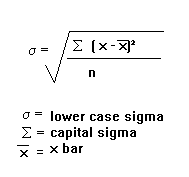MathsGee is Zero-Rated (You do not need data to access) on: Telkom |Dimension Data | Rain | MWEB

2 like 0 dislike
6,653 views
The variance of a sample of 169 observations equals 576. The standard deviation of the sample equals:
| 6,653 views

4 like 0 dislike

The standard deviation is the measure of how the data differs from the mean. The equation is as follows:The equation inside the square root is the equation for the variance.

There for the answer is the 24.

by Wooden (624 points)
selected by
0 like 0 dislike
The standard deviation of the sample equals 24. The square root of 576.
by Wooden (484 points)
0 like 0 dislike
standard deviation is the squre root of a given sample space which is 24 at this case
by Wooden (428 points)

2 like 0 dislike